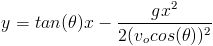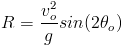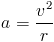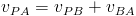# Summary of Chapter 4: Motion in More than One Dimention

Projectile MotionA projectile is an object which is launched at some initial velocity and some angle to the horizontal. Projectiles experience only free fall acceleration. the path of a projectile can be determined using the kinematics equations provided in chapter 2, by separating x and y components of position, velocity and acceleration. the trajectory path of a particle in projectile motion that is parabolic can be determined by:If the initial position in x and y is zero, the horizontal range (R, the horizonal distance traveled by the particle) is given by:Uniform Circular Motion If a particle travels along a circle of radius r and velocity v, there must be a centripetal acceleration directed at the center of the circle given by:The time for this particle to travel around the cirlce is the period which is given by:Relative Motion When two Frames of refrence A and B are moving at diffent constant velocities, the movement of particle P differs between the two refrence frames. The velocities are given by:The observed accelerations are the same:Return to top
All information and equations are taken from and credited to Jearl Walker's Fundamental Physics.